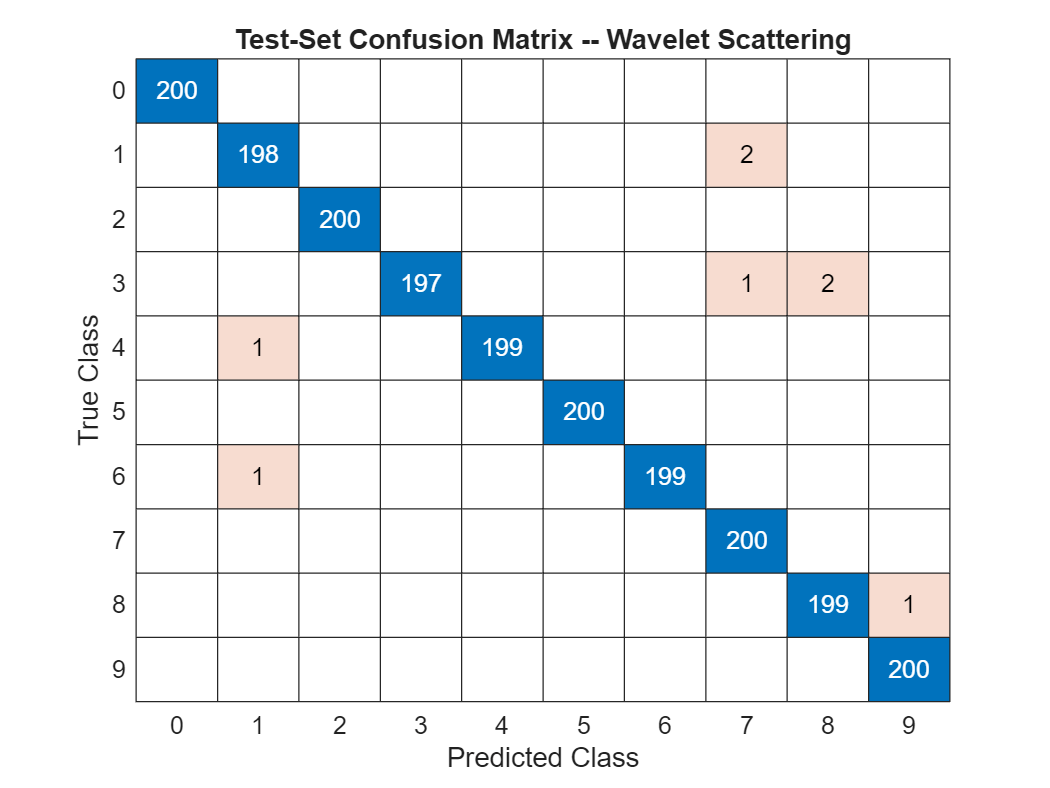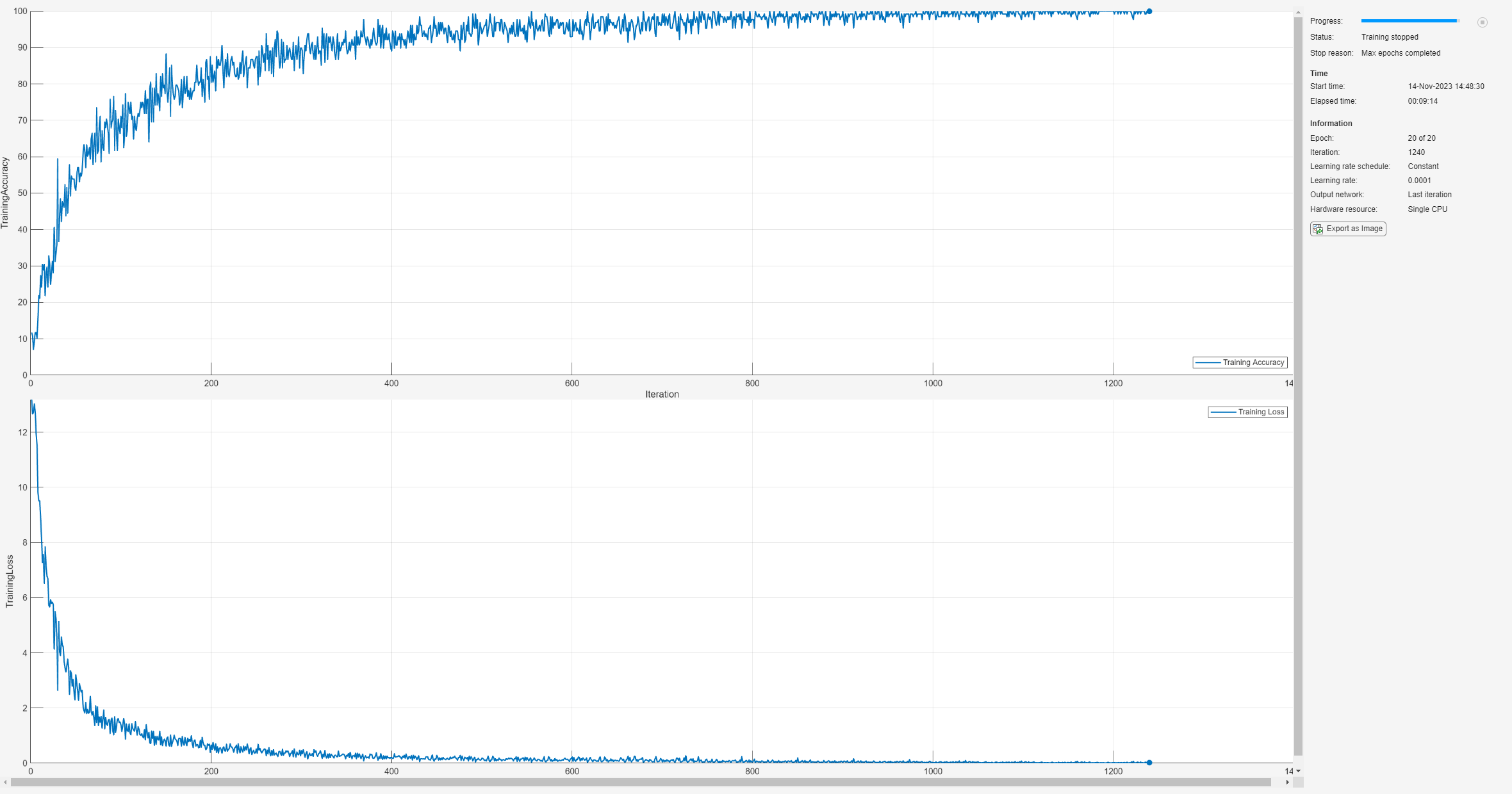Documentation

## Digit Classification with Wavelet Scattering

This example shows how to use wavelet scattering for image classification. This example requires Wavelet Toolbox™, Deep Learning Toolbox™, and Parallel Computing Toolbox™.

For classification problems, it is often useful to map the data into some alternative representation which discards irrelevant information while retaining the discriminative properties of each class. Wavelet image scattering constructs low-variance representations of images which are insensitive to translations and small deformations. Because translations and small deformations in the image do not affect class membership, scattering transform coefficients provide features from which you can build robust classification models.

Wavelet scattering works by cascading the image through a series of wavelet transforms, nonlinearities, and averaging . The result of this deep feature extraction is that images in the same class are moved closer to each other in the scattering transform representation, while images belonging to different classes are moved farther apart. While the wavelet scattering transform has a number of architectural similarities with deep convolutional neural networks, including convolution operators, nonlinearities, and averaging, the filters in a scattering transform are pre-defined and fixed.

### Digit Images

The dataset used in this example contains 10,000 synthetic images of digits from 0 to 9. The images are generated by applying random transformations to images of those digits created with different fonts. Each digit image is 28-by-28 pixels. The dataset contains an equal number of images per category. Use the `imageDataStore` to read the images.

```digitDatasetPath = fullfile(matlabroot,'toolbox','nnet','nndemos','nndatasets','DigitDataset'); Imds = imageDatastore(digitDatasetPath,'IncludeSubfolders',true, 'LabelSource','foldernames');```

Randomly select and plot 20 images from the dataset.

```figure numImages = 10000; rng(100); perm = randperm(numImages,20); for np = 1:20 subplot(4,5,np); imshow(Imds.Files{perm(np)}); end```You can see that the 8's in rows 1, 2, 3, and 4 exhibit considerable variability while all being identifiable as an 8. The same is true of the other repeated digits in the sample. This is consistent with natural handwriting where any digit differs non-trivially between individuals and even within the same individual's handwriting with respect to translation, rotation, and other small deformations. Using wavelet scattering, we hope to build representations of these digits which obscure this irrelevant variability.

### Wavelet Image Scattering Feature Extraction

The synthetic images are 28-by-28. Create a wavelet image scattering framework and set the invariance scale to equal the size of the image. Set the number of rotations to 8 in each of the two wavelet scattering filter banks. The construction of the wavelet scattering framework requires that we set only two hyperparameters: the `InvarianceScale` and `NumRotations.`

```sf = waveletScattering2('ImageSize',[28 28],'InvarianceScale',28, ... 'NumRotations',[8 8]);```

This example makes use of MATLAB™'s parallel processing capability through the `tall `array interface`. `You can start the parallel pool if one is not currently running with the following code. Alternatively, the first time you create a `tall `array, the parallel pool is created.` `

```if isempty(gcp) parpool; end```

For reproducibility, set the random number generator. Shuffle the files of the `imageDatastore` and split the 10,000 images into two sets, one for training and one held-out set for testing. Allocate 80% of the data, or 8,000 images, to the training set and hold out the remaining 2,000 images for testing. Create `tall` arrays from the training and test datasets. Use the helper function, `helperScatImages`, to create feature vectors from the scattering transform coefficients. `helperScatImages` obtains the log of the scattering transform feature matrix as well as the mean along both the row and column dimensions of each image. For each image in this example, this results in a 217-by-1 feature vector.

```rng(10); Imds = shuffle(Imds); [trainImds,testImds] = splitEachLabel(Imds,0.8); Ttrain = tall(trainImds); Ttest = tall(testImds); trainfeatures = cellfun(@(x)helperScatImages(sf,x),Ttrain,'UniformOutput',false); testfeatures = cellfun(@(x)helperScatImages(sf,x),Ttest,'UniformOutput',false);```

Use `tall`'s `gather` capability to concatenate all the training and test features.

`Trainf = gather(trainfeatures);`
```Evaluating tall expression using the Parallel Pool 'local': - Pass 1 of 1: Completed in 5 min 5 sec Evaluation completed in 5 min 5 sec ```
```trainfeatures = cat(2,Trainf{:}); Testf = gather(testfeatures);```
```Evaluating tall expression using the Parallel Pool 'local': - Pass 1 of 1: Completed in 1 min 13 sec Evaluation completed in 1 min 13 sec ```
`testfeatures = cat(2,Testf{:});`

The previous code results in two matrices with row dimensions 217 and column dimension equal to the number of images in the training and test sets respectively. Accordingly, each column is a feature vector for its corresponding image. Note that the original images contained 784 elements. Accordingly, the scattering coefficients represent an approximate 4-fold reduction in the size of each image.

### PCA Model and Prediction

This example constructs a simple classifier based on the principal components of the scattering feature vectors for each class. The classifier is implemented in the functions `helperPCAModel` and `helperPCAClassifier`. `helperPCAModel` determines the principal components for each digit class based on the scattering features. `helperPCAClassifier` classifies the held-out test data by finding the closest match (best projection) between the principal components of each test feature vector with the training set and assigning the class accordingly.

```model = helperPCAModel(trainfeatures,30,trainImds.Labels); predlabels = helperPCAClassifier(testfeatures,model);```

After constructing the model and classifying the test set, determine the accuracy of the test set classification.

`accuracy = sum(testImds.Labels == predlabels)./numel(testImds.Labels)*100`
```accuracy = 99.6000 ```

We have achieved 99.6% correct classification of the test data. Plot the confusion matrix to see how the 2,000 test images have been classified. There are 200 examples in the test set for each of the 10 classes.

```figure; confusionchart(testImds.Labels,predlabels); title('Test-Set Confusion Matrix -- Wavelet Scattering')```### CNN

In this section, we train a simple convolutional neural network (CNN) to recognize digits. Construct the CNN to consist of a convolution layer with 20 5-by-5 filters with 1-by-1 strides. Follow the convolution layer with a RELU activation and max pooling layer. Use a fully connected layer, followed by a softmax layer to normalize the output of the fully connected layer to probabilities. Use a cross entropy loss function for learning.

```imageSize = [28 28 1]; layers = [ ... imageInputLayer([28 28 1]) convolution2dLayer(5,20) reluLayer maxPooling2dLayer(2,'Stride',2) fullyConnectedLayer(10) softmaxLayer classificationLayer];```

Use stochastic gradient descent with momentum and a learning rate of 0.0001 for training. Set the maximum number of epochs to 20. For reproducibility, set the `ExecutionEnvironment `to '`cpu'`.

```options = trainingOptions('sgdm', ... 'MaxEpochs',20,... 'InitialLearnRate',1e-4, ... 'Verbose',false, ... 'Plots','training-progress','ExecutionEnvironment','cpu');```

Train the network. For training and testing we use the same data sets used in the scattering transform.

```reset(trainImds); reset(testImds); net = trainNetwork(trainImds,layers,options);```By the end of training, the CNN is performing near 100% on the training set. Use the trained network to make predictions on the held-out test set.

```YPred = classify(net,testImds,'ExecutionEnvironment','cpu'); DCNNaccuracy = sum(YPred == testImds.Labels)/numel(YPred)*100```
```DCNNaccuracy = 95.1500 ```

The simple CNN has achieved 95.15% correct classification on the held-out test set. Plot the confusion chart for the CNN.

```figure; confusionchart(testImds.Labels,YPred); title('Test-Set Confusion Chart -- CNN')```### Summary

This example used wavelet image scattering to create low-variance representations of digit images for classification. Using the scattering transform with fixed filter weights and a simple principal components classifier, we achieved 99.6% correct classification on a held-out test set. With a simple CNN in which the filters are learned, we achieved 95.15% correct. This example is not intended as a direct comparison of the scattering transform and CNNs. There are multiple hyperparameter and architectural changes you can make in each case, which significantly affect the results. The goal of this example was simply to demonstrate the potential of deep feature extractors like the wavelet scattering transform to produce robust representations of data for learning.

### REFERENCES

 Bruna, J. and Mallat, S. (2013) "Invariant scattering convolutional networks", IEEE Transactions on Pattern Analysis and Machine Intelligence, 35(8), pp. 1872-1886.

 Mallat, S. (2012) "Group invariant scattering", Communications on Pure and Applied Mathematics, 65, pp. 1331-1398.

 Sifre, L. and Mallat, S. (2013). "Rotation, scaling and deformation invariant scattering for texture discrimination", Proceedings / CVPR, IEEE Computer Society Conference on Computer Vision and Pattern Recognition. IEEE Computer Society Conference on Computer Vision and Pattern Recognition. 1233-1240. 10.1109/CVPR.2013.163.